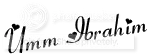# Tag Archives: reviewing decimals

## Review Decimals: TenthsIt’s been a while since my fifth grader has studied decimals and I haven’t kept up with review like I would like. Today we came to decimals for the first time this grade and he really needed review.  So instead of doing the lesson as was outlined in the book, I stopped and went back to basics.  I made up a little review routine to try to give him lots of practice, insha Allah.

The book hits the tenths, hundredths, and thousandths all at one time, but we’re taking it back to the beginning and just focusing on one place at a time. I want to make sure that he understands the value of each digit in a decimal (e.g. .516, the value of the five is .5, the value of the 1 is .01, and the value of the 6 is .006.  At first he was having trouble with this (and likewise with writing whole numbers in expanded form) and so I wanted to make sure that he understood the VALUE of each digit in a decimal.  He seemed like he kind of got the hang of it, but I want to make sure, so that’s why were taking a short detour from the book.  (Normally, that would be an alarm for me as when I stop something, its hard to get back without me changing books, but I really like this book, so insha Allah we’ll stick with it, but I want him to be firm on the concepts before moving on.

So, below I share my little review routine that I wrote up for practicing decimals in the tenths.  Tomorrow, insha Allah, we’ll  hit the hundredths and then the next day the thousandths following this same practice pattern, insha Allah.

 0.4 0.6 0.1 0.9 0.8 0.5 0.3 0.2 0.7

### WRITE the fraction, word form and picture for each decimal.

 Fraction Decimal Word Form 4/10 0.4 four tenths 0.6

In the printout (see end of post) , there is space to color in the appropriate amount of blocks out of 10.

### What is the value of each digit?

 0.5 0.7 0.4 0.1 0.3 0.2 0.8 0.9 0.6

### Listen then Write

Teacher: See note on next page for words to dictate.

Listen/Write Instructions: (Dictate these words): decimal, fraction, each of the tenths (one tenth nine tenths randomly)

Then, as we encounter concepts in the book, I make up a little data bank of questions:

Review Data Bank Questions

 Spell decimal d-e-c-i-m-a-l What is a decimal? A fraction whose denominator is 10 or a power of 10. Given a decimal (in the tenths), read it Given a decimal in the tenths orally, write it in fraction and decimal form Write the word form for the decimal I say (in the tenths) Draw a picture to show the decimal (in the tenths) I say

Ideally, I like to make cards, but as its time consuming, I tend to just make a list like this with the question/activity on the left and the answer/response on the right.

So insha Allah, I’ll follow with similar activities for hundredths and thousandths (well at least that’s the plan).

Here’s the printable for this:Comments Off on Review Decimals: Tenths

Posted by on November 16, 2009 in Math: Decimals/Fractions/Percent